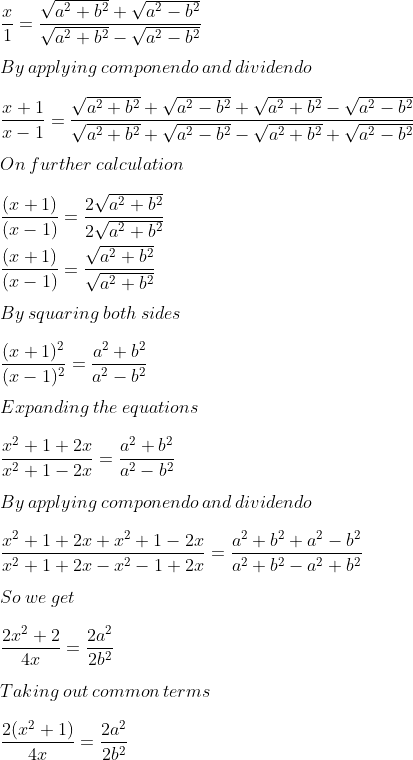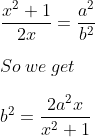Guru

# Given x=root(a2+b2) +root(a2−b2) /(root (a2+b2) −root(a2−b2) Use componendo and dividendo to prove that b²=2a²x/x²+1

• 0

This is a basic question from ML aggarwal book of class 10th, chapter 7, ratio and proportion., ICSE board

We have been given the value of x and we have to prove the validity of the given equation by using componendo dividendo.

Question 15, 7.3

Share